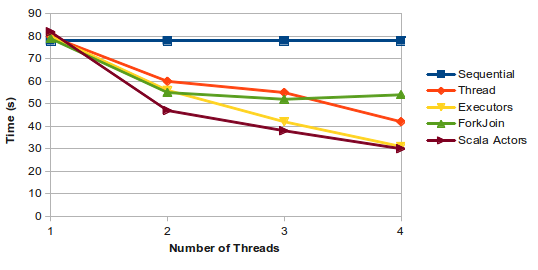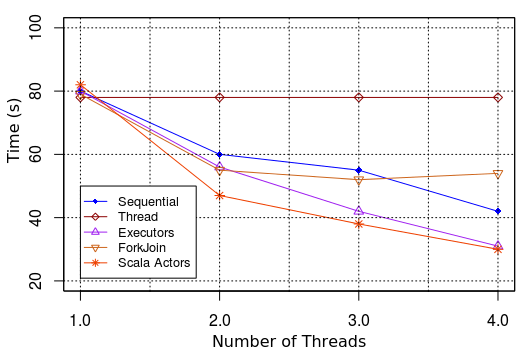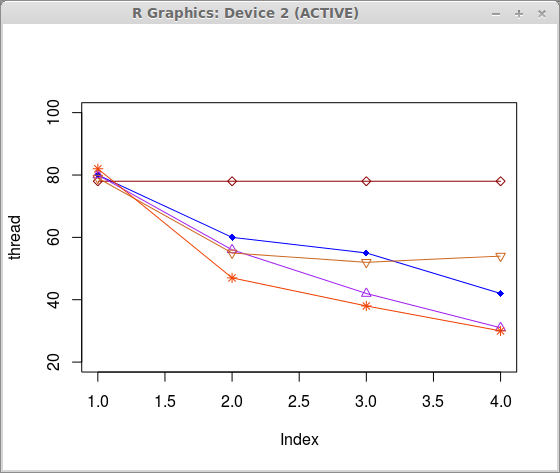Hi there.

As you may know, I’m a graduate student and part of my work is spent on writing papers. And, frequently, these papers contains a lot of charts. As long as I’m a graduate student, I’m very disapointed with the charts that open-office plots. Specially, the line charts. It is kinda a four-years-old-children playing with their paint and brush. Do not believe? Take a look.It is terrible.

So, yesterday I really decided to revert this game. Surfing on the internet, I read some people writing that R is outstanding to ploting charts, which I had serious doubts, mainly because I have been using R for a long time. But, most of the time is to plot boxplot, or to use few functions such as `summary`, `sd`, `cor`, among more two or three. Not less, not more. In my (naive) imagination, R should had a function like `plotanincrediblechart`, and I only should pass the data array. But R does not have this function. In fact, R has a number of functions, and, when you play with they together, you can get a nice chart like the this one.Much better, uh?

So, how can you do this?

# First

Let’s start by initializing your data array. If you do not have basic knowledge in R, this article is a good start point.

``````seq <- c(78, 78, 78, 78)
thread <- c(80, 60, 55, 42)
executors <- c(80, 56, 42, 31)
forkJoin <- c(79, 55, 52, 54)
actors <-c(82, 47, 38, 30)
``````

# Second

Create a simple line chart with one of your data set using the `plot` function. In this case, I used the `thread` var.

``````plot(thread, type="o", col="blue", pch=18, ylim=c(20, 100))
``````

In this function, we passed the `thread` var and more four arguments. They are:

• `type`: the plot type;
• `col`: the line color (see here to see all colors avaiable in R);
• `pch`: the vector of plotting characters or symbols;
• `ylim`: the y limits of the plot;

There are several other parameters that you can use in your chart. If you have the time, take a lot at the official documentation.

Now, we have to add the remaining data. To do so, we will use another function called `lines`. In this example, we pass five arguments to this function. Note that these arguments are the same ones that we have passed to the `plot` function. Therefore, for each new vector that you want to plot, you have to add a new line, such as follows:

``````lines(seq, type="o", pch=23, lty=1, col="darkred")
lines(executors, type="o", pch=24, lty=1, col="purple")
lines(forkJoin, type="o", pch=25, lty=1, col="chocolate3")
lines(actors, type="o", pch=8, lty=1, col="orangered2 ")</code></pre>
``````

For the moment, your chart may looks like this one:Great! Our chart is becoming professional. My mon will be proud of me.

# Third

The third part is to add both vertical and horizontal lines.

``````abline(v=1,col=1,lty=3)
abline(v=1.5,col=1,lty=3)
abline(v=2,col=1,lty=3)
abline(v=2.5,col=1,lty=3)
abline(v=3,col=1,lty=3)
abline(v=3.5,col=1,lty=3)
abline(v=4,col=1,lty=3)

abline(h=100,col=1,lty=3)
abline(h=80,col=1,lty=3)
abline(h=60,col=1,lty=3)
abline(h=40,col=1,lty=3)
abline(h=20,col=1,lty=3)
``````

Note that, we first add the vertical lines, and then, the horizontal lines. We differ each other by the first parameter (`v` for vertical lines and `h` for horizontal lines). The second parameter, as you might guess, is the color of the line, and the last one is the line type. Line types can either be specified as an integer (0=blank, 1=solid, …) or as one of the character strings “blank”, “solid”, etc.

# Fourth

The fourth and finally part is to plot a box with the lines information. To this, you will need two functions: `box` and `legend`. I think the name of these functions are meaningful. But, the `legend` function receives several parameters.

``````box()
labels <- c("Sequential","Thread", "Executors", "ForkJoin", "Scala Actors")
colors <- c("blue","darkred", "purple", "chocolate3", "orangered2")
pch <- c(18,23,24,25,8)

legend(1.0, 50, labels, cex=0.8, col=colors, pch=phc, lty=1,bg = "white");
``````

And you’ll probably see a very similar chart like the second topmost in this post. All code is pasted here. And, that is it! :-)

See you later alligator!

Categories:

Updated: